# 利用MATLAB实现正态参数估计及分布检验

• 三道题的分析与代码实现
• 实验值与理论值的比较
• 完整代码附录

### 理论分析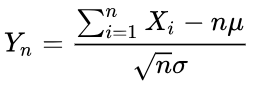### MATLAB代码实现详解

function result = my_rand()
below_zeros = -rand(1,50);
above_zeros = rand(1,50);
rand_sum = sum(below_zeros) + sum(above_zeros);
total = 100;
result = (rand_sum - total*0.5) / (10*total*total/12);
end

rand_x = [];
for  time = 1:1000
rand_x = [rand_x,my_rand()];
end

### 理论分析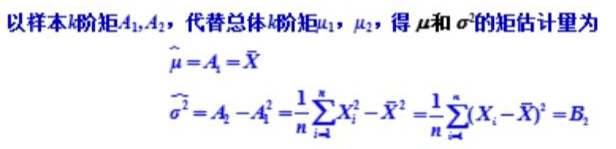### MATLAB代码实现详解

index = [];
for time = 1:1000
index = [index,time]; %产生1~1000的下标数组
end
rand_index = randperm(1000,100); %产生1~1000的100个不重复的随机下标
sample = [];
for time = 1:100
sample = [sample,rand_x(rand_index(time))];
end

predict_ex = sum(sample)/100; %由样本估计出的均值
sample1 = sample.^2; %将sample数组中的每一个值都平方
square_sum = sum(sample1);
predict_dx = square_sum/100 - predict_ex*predict_ex;
%由样本估计的方差

### 理论分析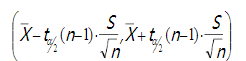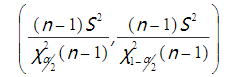### MATLAB代码实现详解

left_ex = predict_ex - 1.9842*sqrt(predict_dx)/10;
%均值置信区间左侧
right_ex = predict_ex + 1.9842*sqrt(predict_dx)/10;
%均值置信区间右侧
left_dx = (99*predict_dx)/128.422;
%方差置信区间左侧
right_dx = (99*predict_dx)/73.361;
%方差置信区间右侧

### 实验值与理论值比较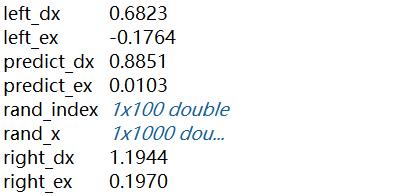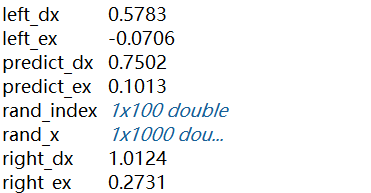### 完整代码附录

%产生标准正态分布，该部分涉及的my_rand()函数定义在最后
rand_x = [];
for time = 1:1000
rand_x = [rand_x,my_rand()];
end
%样本抽取
index = [];
for time = 1:1000
index = [index,time];
end
rand_index = randperm(1000,100);
sample = [];
for time = 1:100
sample = [sample,rand_x(rand_index(time))];
end
%参数估计
predict_ex = sum(sample)/100;
sample1 = sample.^2;
square_sum = sum(sample1);
predict_dx = square_sum/100 - predict_ex*predict_ex;
%求置信区间
left_ex = predict_ex - 1.9842*sqrt(predict_dx)/10;
right_ex = predict_ex + 1.9842*sqrt(predict_dx)/10;
left_dx = (99*predict_dx)/128.422;
right_dx = (99*predict_dx)/73.361;
%展示结果数据
disp(left_ex);
disp(right_ex);
disp(left_dx);
disp(right_dx);
disp(predict_ex);
disp(predict_dx);
%自定义函数用于产生单个正态分布随机数
function result = my_rand()
below_zeros = -rand(1,50);
above_zeros = rand(1,50);
rand_sum = sum(below_zeros) + sum(above_zeros);
total = 100;
result = (rand_sum - total*0) / (10*4/12);
end04-123万+
05-283万+
08-141万+
02-211万+
12-04
01-03229
09-304080
04-102万+
01-27781
02-191万+
01-281万+
05-02896
02-198204
04-131万+
08-191万+
12-131万+
03-012613
04-189519